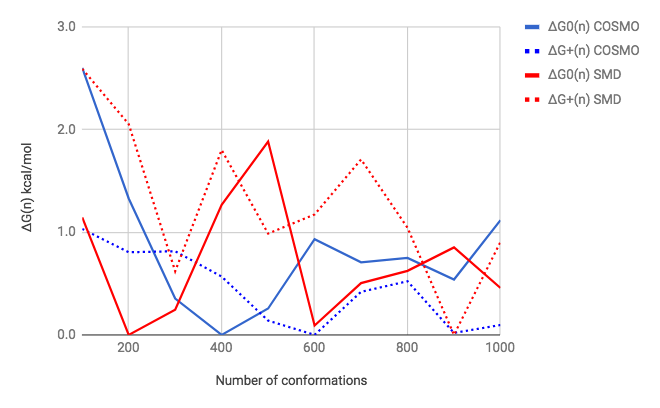## Wednesday, February 14, 2018

### Predicting pKa values using PM3 - conformer search part 3This is a follow up to this post, but now we (that is Mads) use up to 1000 structures. To summarise: we use RDKit to generate $n$ starting conformations of neutral ("0") and protonated ("+") acebutolol and minimise them in aqueous solution using PM3/COSMO (MOPAC) and PM3/SMD /GAMESS) for a maximum of 200 steps.

The plot shows the lowest energy structure relative to the lowest energy found among all values of $n$. For example, the lowest PM3/COSMO value for the neutral form is found when generating 400 starting geometries and using 1000 starting structure, the lowest energy found is 1.1 kcal/mol higher.

I was a bit worried about the numerical stability of the COSMO and SMD methods, i.e. that the $n$ = 400 structure was generated in the $n$ = 1000 run, but had a higher energy for some reason. The figure above shows the lowest energy structure found ($n$ = 400) in purple and the structure most closely resembling it in the $n$ = 1000 search in green. So the $n$ = 400 structure was not generated in the $n$ = 1000 run.

Acebutolol has 12 rotateable bonds and roughly $3^{12}$ = 500,000 possible rotamers and RDKit samples these randomly. So, on hindsight, it is not surprising that $n$ = 400 and $n$ = 1000 randomly chosen samples do not both contain the lowest energy structure.

Incredibly (at least to me), the green structure is 1.7 kcal/mol higher in energy than the green. But, further optimisation of the green structure does not change energy, nor does changing the optimiser to EF instead of the default. I even rotated the molecule and restarted to the optimisation to test the robustness of the COSMO grid. But, no, this energy difference due to what I think is a minor strutural difference appears to be real. This means that the conformational PES is quite rugged, i.e. small structural changes can result in relatively big energy changes.

As it stands, pKa-predictions using PM3/COMSO or SMD (where I use $n$ > 100) will have a ca 1 pKa unit random error due to the conformational search and increasing $n$ is not really going to decrease that very much.

I'm not sure what to do about that.  We might try combining several low-$n$ conformational searches, with different random seeds. Alternatively, we may have to implement some sort of directed search, e.g. a genetic algorithm but that is going to be quite expensive for an SQM based method.

Alternatively, we have to pick the structures we use based on an MM screen where we can do a more thorough search but then we really need a MM/continuum solvation method interface.  If you have other ideas I'd be happy to hear about it.

Let me end with a challenge. Can you make a robust method that can find a PM3/COSMO energy equal to or lower than -183.01 kcal/mol for neutral acebutolol? And that is converged with respect to starting geometry and number of conformers tested? The MOPAC keywords are

pm3 eps=78.4 charge=0 cycles=200